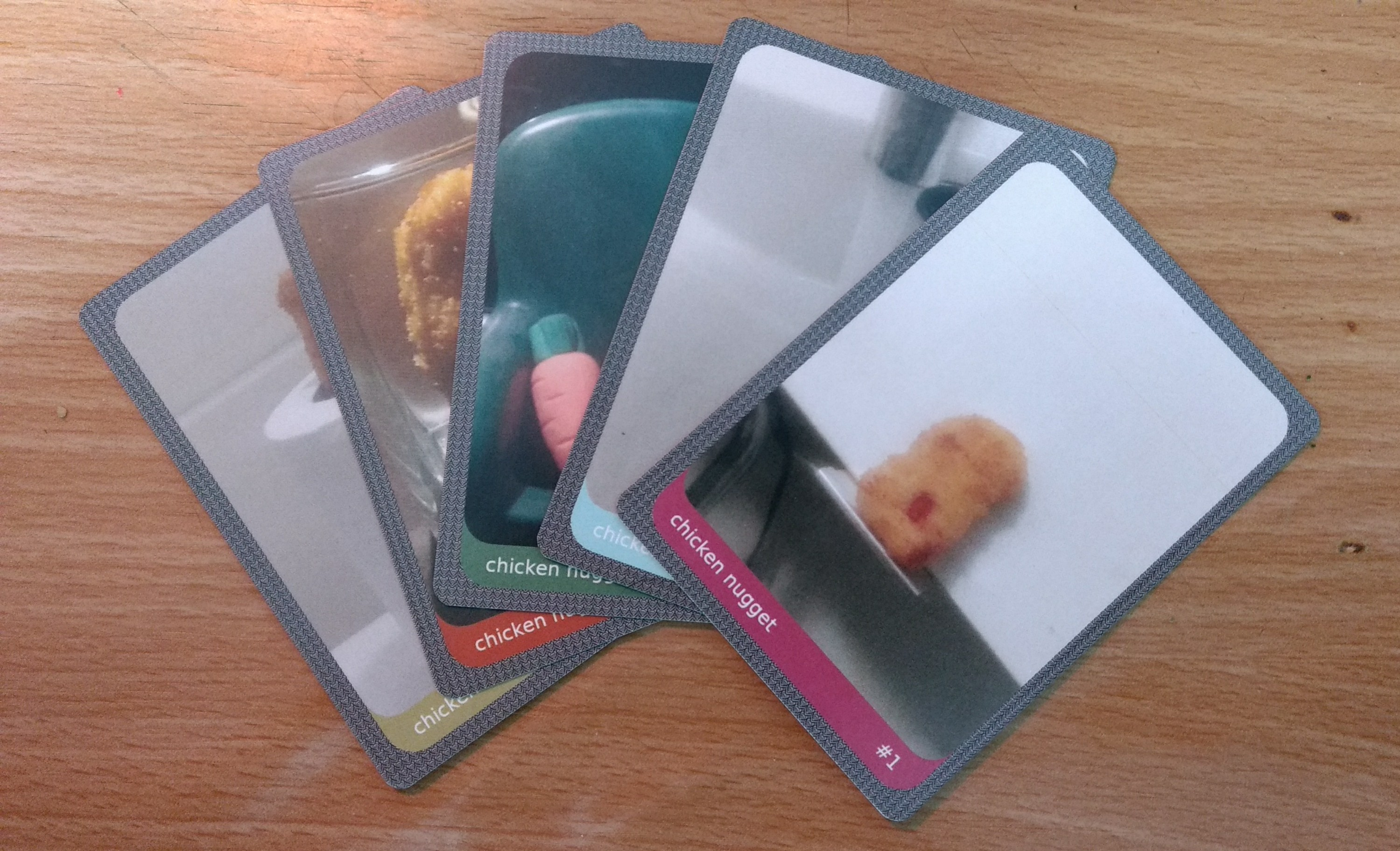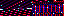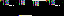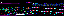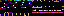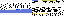EVR.Level.Prompt = function(level)
{
this.level = level;
this.initialize();
this.append();
}
EVR.Level.Prompt.prototype = new EVR.Prompt;
EVR.Level.Prompt.prototype.initialize = function()
{
var container = this.level.container;
var text = LEVEL_START_PROMPT_TEXT;
var color = LEVEL_PROMPT_COLOR;
var size = LEVEL_PROMPT_SIZE;
var background = LEVEL_PROMPT_BACKGROUND;
var depth = LEVEL_PROMPT_DEPTH;
EVR.Prompt.call(
this, container, text, color, size, background, depth, null, offset);
}
EVR.Level.Prompt.prototype.set_finish_text = function()
{
var level = this.level;
var text = LEVEL_FINISHED_PROMPT_TEXT;
if (level.id == LEVEL_LIMIT && level.is_clear())
{
text += ", " + LEVEL_VIEW_ENDING_PROMPT_TEXT;
}
else if (level.next_level_is_available())
{
text = LEVEL_CLEAR_PROMPT_TEXT;
}
this.set_text(text);
}
EVR.Level.Prompt.prototype.toggle = function()
{
var attached = this.attached;
attached && this.remove();
!attached && this.append();
}
EVR.Level.Prompt.prototype.toString = function()
{
return "[object EVR.Level.Prompt]";
}
EVR.include("level/cheers/marquee/Marquee.js");
EVR.Level.Cheers = function(level)
{
EVR.Graphic.call(this, level.container, null, null, ALIGN_TOP);
EVR.Animation.call(this, CHEERS_ANIMATION_RATE);
this.level = level;
this.cheer_texts = CHEERS_TEXTS;
this.boost_bounds = [CHEERS_MIN_BOOST_LENGTH, CHEERS_MAX_BOOST_LENGTH];
this.drag_threshold = CHEERS_DRAG_THRESHOLD;
this.step = CHEERS_SCROLL_STEP;
this.delay = CHEERS_SCROLL_DELAY;
this.last_time = null;
this.boost_length = null;
this.drag_length = null;
this.scrolling = false;
this.setAttributes();
this.append();
this.background = new EVR.Level.Cheers.Marquee(
this.foreground = new EVR.Level.Cheers.Marquee(
this, null, null, CHEERS_FOREGROUND_Z_INDEX, CHEERS_FOREGROUND_OPACITY);
}
EVR.Level.Cheers.prototype = new EVR.Graphic;
EVR.inherit(EVR.Level.Cheers, EVR.Animation);
EVR.Level.Cheers.prototype.setAttributes = function()
{
this.set_proportions(CHEERS_MARQUEE_WIDTH, CHEERS_MARQUEE_HEIGHT);
this.place(0, CHEERS_MARQUEE_OFFSET);
this.css.overflow = "hidden";
}
EVR.Level.Cheers.prototype.scroll = function()
{
var marquee_length = this.calculateScrollLength();
var width = this.get_dimensions();
var cutoff = -marquee_length + width * (1 - CHEERS_SCROLL_PADDING);
this.scrolling = true;
this.play(null, this.delay, cutoff);
}
EVR.Level.Cheers.prototype.calculateScrollLength = function()
{
var length = 0;
var glyphs = this.foreground.glyphs;
for (var ii = 0; ii < glyphs.length; ii++)
{
length += glyphs[ii].offsetWidth;
}
return length;
}
EVR.Level.Cheers.prototype.sequence = function(cutoff)
{
var step = this.step;
var foreground = this.foreground;
var background = this.background;
var foreground_x = foreground.get_coordinates() - step;
var background_x = background.get_coordinates() - step;
if (background_x < cutoff)
{
foreground_x = 0;
background_x = 0;
this.stop();
this.play(null, this.delay, cutoff);
}
foreground.set_coordinates([foreground_x]);
background.set_coordinates([background_x]);
}
EVR.Level.Cheers.prototype.update = function(sprinting, speed)
{
if (this.level.practice)
{
return;
}
var time = this.level.clock.time.get();
var interval = time - this.last_time;
this.last_time = time;
this.updateBoostTimer(sprinting, speed, interval);
this.updateDragTimer(sprinting, speed, interval);
}
EVR.Level.Cheers.prototype.updateBoostTimer =
function(sprinting, speed, interval)
{
if (sprinting && speed == PATH_SPRINT_SPEED)
{
this.boost_length += interval;
if (this.boost_length >= this.boost_bounds)
{
this.boost_length = 0;
}
}
else if (this.boost_length != 0)
{
this.boost_length = 0;
}
}
{
var length = this.boost_length;
var bounds = this.boost_bounds;
if (length >= bounds)
{
this.drag_length = 0;
if (length > bounds)
{
this.boost_length = bounds;
}
var cheer = this.determineCheerText();
}
}
EVR.Level.Cheers.prototype.determineCheerText = function()
{
var bounds = this.boost_bounds;
var range = bounds - bounds;
var offset = this.boost_length - bounds;
var texts = this.cheer_texts;
var index = Math.round((texts.length - 1) * (offset / range));
return texts[index];
}
EVR.Level.Cheers.prototype.updateDragTimer =
function(sprinting, speed, interval)
{
if (speed < PATH_INITIAL_SPEED || (speed < PATH_SPRINT_SPEED && sprinting))
{
this.drag_length += interval;
if (this.drag_length >= this.drag_threshold)
{
this.drag_length = 0;
}
}
}
{
}
EVR.Level.Cheers.prototype.draw = function()
{
EVR.Graphic.prototype.draw.call(this);
if (!!this.foreground)
{
this.foreground.draw();
this.background.draw();
}
if (this.scrolling)
{
this.stop();
this.scroll();
}
}
EVR.Level.Cheers.prototype.remove = function()
{
EVR.Graphic.prototype.remove.call(this);
if (!!this.foreground)
{
this.foreground.remove();
this.background.remove();
}
if (this.scrolling)
{
this.stop();
this.scrolling = false;
}
}
EVR.Level.Cheers.prototype.toString = function()
{
return "[object EVR.Level.Cheers]";
}
54.80.252.84
54.80.252.84
54.80.252.84

March 22, 2020

The chicken nugget business starter kit is now available online! Send me any amount of money through Venmo or PayPal, and I will mail you a package which will enable you to start a chicken nugget business of your own, play a video game any time you want, introduce a new character into your Animal Crossing village, and start collecting the chicken nugget trading cards.

The kit includes:

• jellybean
• instruction manual